# Regular polygons

By Martin McBride, 2022-04-30
Tags: regular polygon equilateral triangle square pentagon hexagon heptagon octagon nonagon decagon hendecagon dodecagon interior angle exterior angle
Categories: gcse geometryA regular polygon is a polygon for which every side is equal and every angle is equal. In this section we will look at some well known regular polygons with 3 to 12 sides. Here is a video:

## Interior and exterior angles of regular polyons

This section gives the formulas for the interior and exterior angles of a polygon.

For a regular polygon, we know that all the interior angles are equal, and also that all the exterior angles are equal. We can use this to show that. for a regular polygon of n sides:

$$exterior = \frac{360°}{n}$$

$$interior = 180° - \frac{360°}{n}$$

## 3 sides - equilateral triangle

A three sided shape, of course, is called a triangle. A regular triangle is called an equilateral triangle, and looks like this: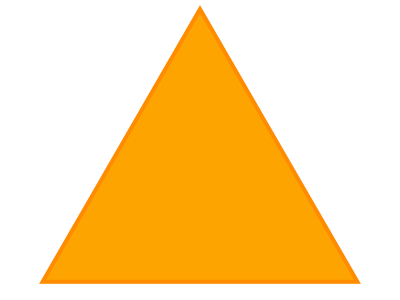Interior angle of a equilateral triangle is 60.0°

Exterior angle of a equilateral triangle is 120.0°

## 4 sides - square

A four sided shape is called a quadrilateral. A regular quadrilaterals are more commonly known as squares: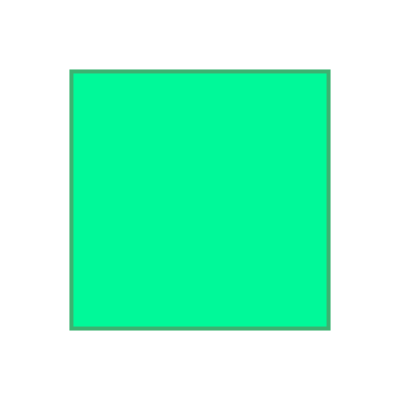Interior angle of a square is 90.0°

Exterior angle of a square is 90.0°

## 5 sides - pentagon

A five sided shape is called a pentagon. A regular pentagon looks like this:Interior angle of a regular pentagon is 108.0°

Exterior angle of a regular pentagon is 72.0°

## 6 sides - hexagon

A six sided shape is called a hexagon. A regular hexagon looks like this: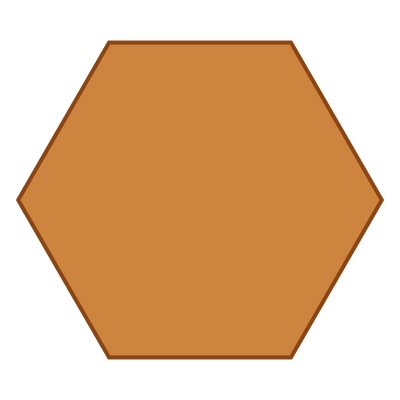Interior angle of a regular hexagon is 120.0°

Exterior angle of a regular hexagon is 60.0°

## 7 sides - heptagon

A seven sided shape is called a heptagon. A regular heptagon looks like this:Interior angle of a regular heptagon is 128.57°

Exterior angle of a regular heptagon is 51.43°

## 8 sides - octagon

A eight sided shape is called an octagon. A regular octagon looks like this: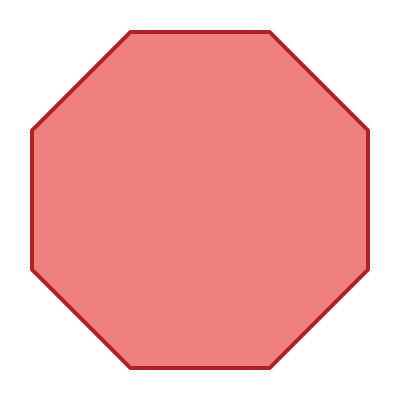Interior angle of a regular octagon is 135.0°

Exterior angle of a regular octagon is 45.0°

## 9 sides - nonagon

A nine sided shape is called a nonagon. A regular nonagon looks like this: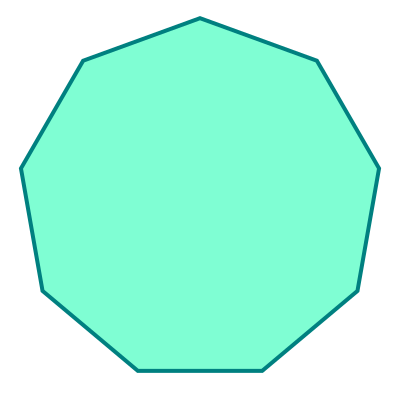Interior angle of a regular nonagon is 140.0°

Exterior angle of a regular nonagon is 40.0°

## 10 sides - decagon

A ten sided shape is called a decagon. A regular decagon looks like this: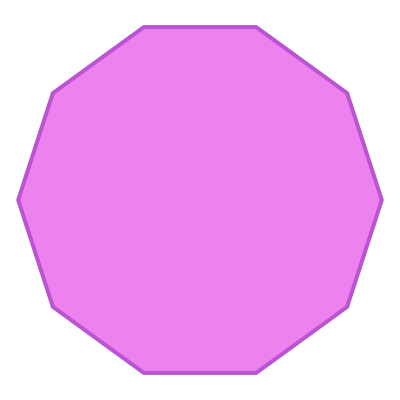Interior angle of a regular decagon is 144.0°

Exterior angle of a regular decagon is 36.0°

## 11 sides - hendecagon

An eleven sided shape is called a hendecagon. A regular hendecagon looks like this: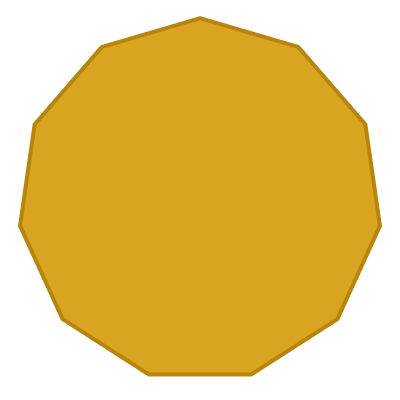Interior angle of a regular hendecagon is 147.27°

Exterior angle of a regular hendecagon is 32.73°

## 12 sides - dodecagon

A twelve sided shape is called a dodecagon. A regular dodecagon looks like this: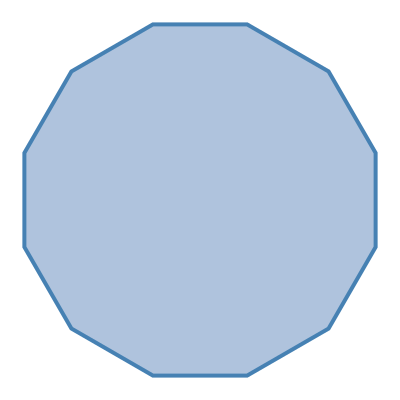Interior angle of a regular dodecagon is 150.0°

Exterior angle of a regular dodecagon is 30.0°

## Polygons with more sides

A polygon can have any number of sides, but most do not have well known special names.

## Irregular polygons

An irregular polygon is a polygon where some of the sides and/or angles are different. Some irregular triangles and quadrilaterals have special names (isosceles triangle, rectangle, parallelogram, etc), but irregular shapes with more than four side usually don't.